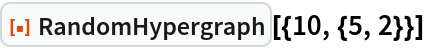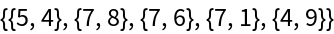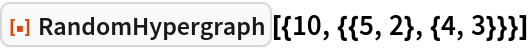#Function Repository Resource:

# RandomHypergraph

Generate a random hypergraph

Contributed by: Stephen Wolfram
 ResourceFunction["RandomHypergraph"][{n,{e,a}}] generates a random hypergraph with e hyperedges of arity a and at most n nodes. ResourceFunction["RandomHypergraph"][{n,{{e1,a1},{e2,a2},…}}] generates a random hypergraph with ei hyperedges of arity ai.

## Examples

### Basic Examples

Generate a hypergraph with 10 nodes and 5 binary hyperedges:

 In:=Out=Generate a hypergraph with 5 binary hyperedges and 4 ternary hyperedges:

 In:=Out=